Скачать презентацию Top-Down Parsing The parse tree is created

362163d4c26befc6e5f93eacb5340c5c.ppt

• Количество слайдов: 31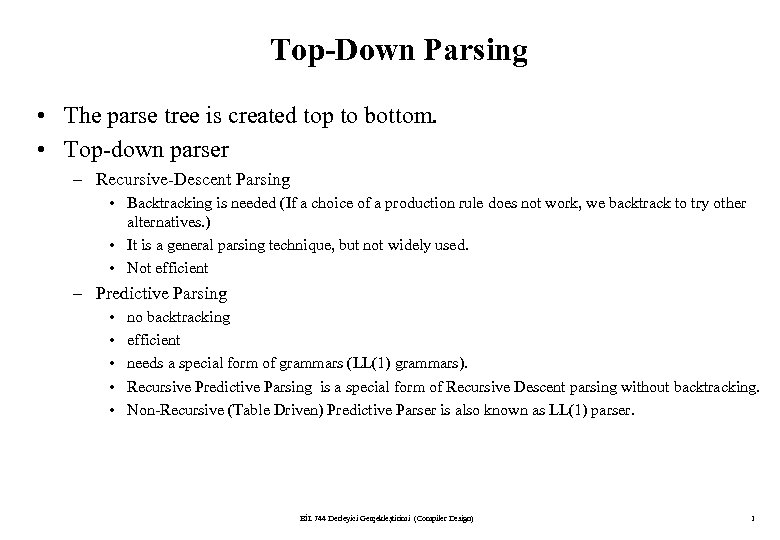Top-Down Parsing • The parse tree is created top to bottom. • Top-down parser – Recursive-Descent Parsing • Backtracking is needed (If a choice of a production rule does not work, we backtrack to try other alternatives. ) • It is a general parsing technique, but not widely used. • Not efficient – Predictive Parsing • • • no backtracking efficient needs a special form of grammars (LL(1) grammars). Recursive Predictive Parsing is a special form of Recursive Descent parsing without backtracking. Non-Recursive (Table Driven) Predictive Parser is also known as LL(1) parser. BİL 744 Derleyici Gerçekleştirimi (Compiler Design) 1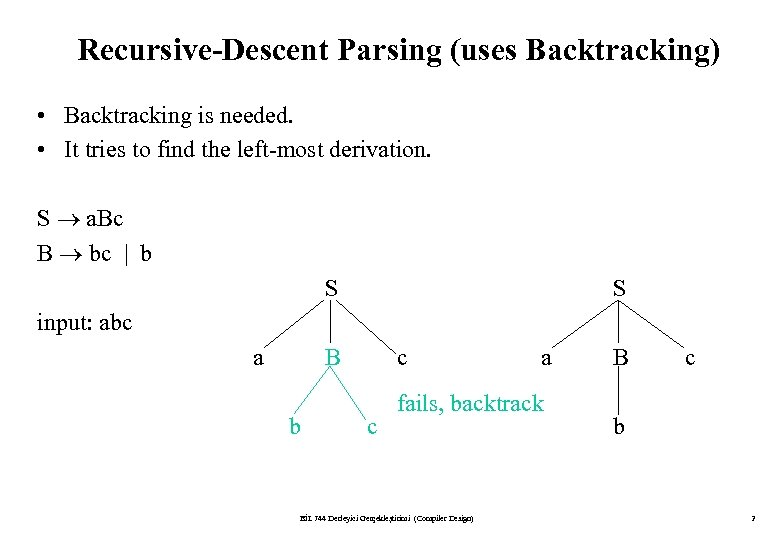Recursive-Descent Parsing (uses Backtracking) • Backtracking is needed. • It tries to find the left-most derivation. S a. Bc B bc | b S S input: abc a B b c c a fails, backtrack BİL 744 Derleyici Gerçekleştirimi (Compiler Design) B c b 2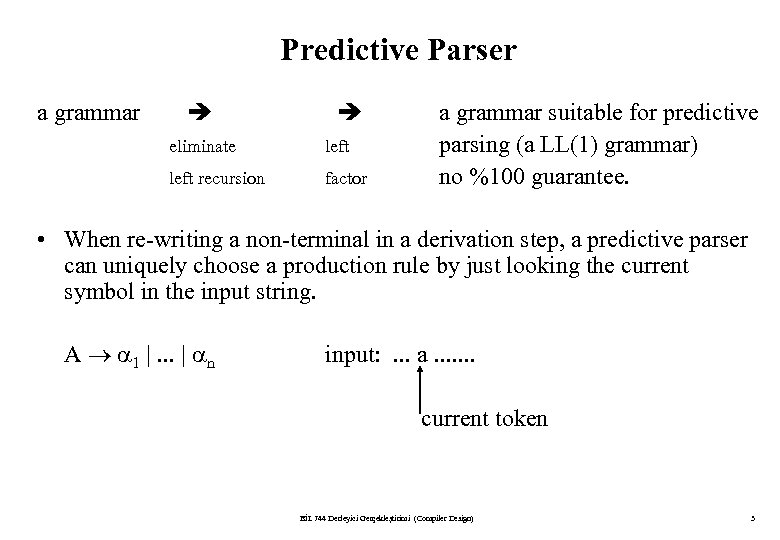Predictive Parser a grammar eliminate left recursion factor a grammar suitable for predictive parsing (a LL(1) grammar) no %100 guarantee. • When re-writing a non-terminal in a derivation step, a predictive parser can uniquely choose a production rule by just looking the current symbol in the input string. A 1 |. . . | n input: . . . a. . . . current token BİL 744 Derleyici Gerçekleştirimi (Compiler Design) 3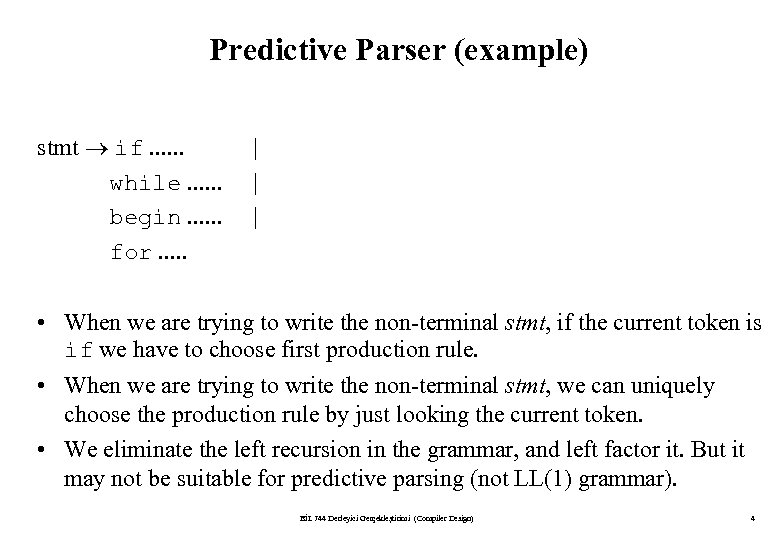Predictive Parser (example) stmt if. . . while. . . begin. . . for. . . | | | • When we are trying to write the non-terminal stmt, if the current token is if we have to choose first production rule. • When we are trying to write the non-terminal stmt, we can uniquely choose the production rule by just looking the current token. • We eliminate the left recursion in the grammar, and left factor it. But it may not be suitable for predictive parsing (not LL(1) grammar). BİL 744 Derleyici Gerçekleştirimi (Compiler Design) 4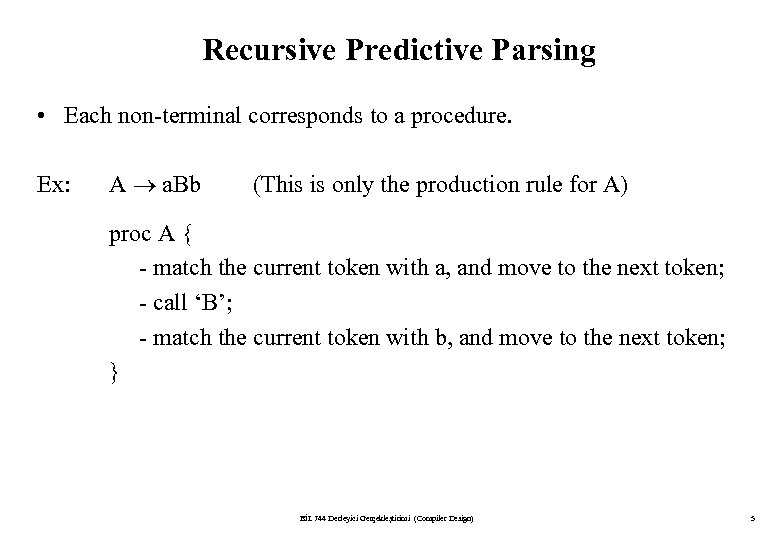Recursive Predictive Parsing • Each non-terminal corresponds to a procedure. Ex: A a. Bb (This is only the production rule for A) proc A { - match the current token with a, and move to the next token; - call ‘B’; - match the current token with b, and move to the next token; } BİL 744 Derleyici Gerçekleştirimi (Compiler Design) 5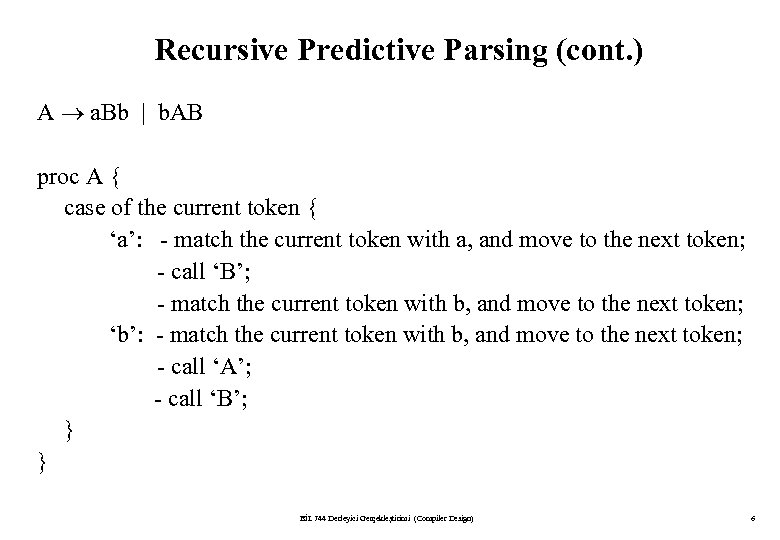Recursive Predictive Parsing (cont. ) A a. Bb | b. AB proc A { case of the current token { ‘a’: - match the current token with a, and move to the next token; - call ‘B’; - match the current token with b, and move to the next token; ‘b’: - match the current token with b, and move to the next token; - call ‘A’; - call ‘B’; } } BİL 744 Derleyici Gerçekleştirimi (Compiler Design) 6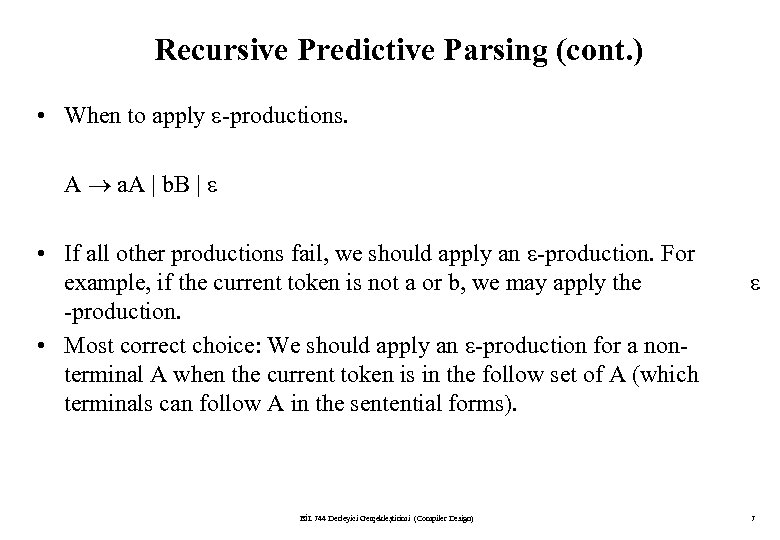Recursive Predictive Parsing (cont. ) • When to apply -productions. A a. A | b. B | • If all other productions fail, we should apply an -production. For example, if the current token is not a or b, we may apply the -production. • Most correct choice: We should apply an -production for a nonterminal A when the current token is in the follow set of A (which terminals can follow A in the sentential forms). BİL 744 Derleyici Gerçekleştirimi (Compiler Design) 7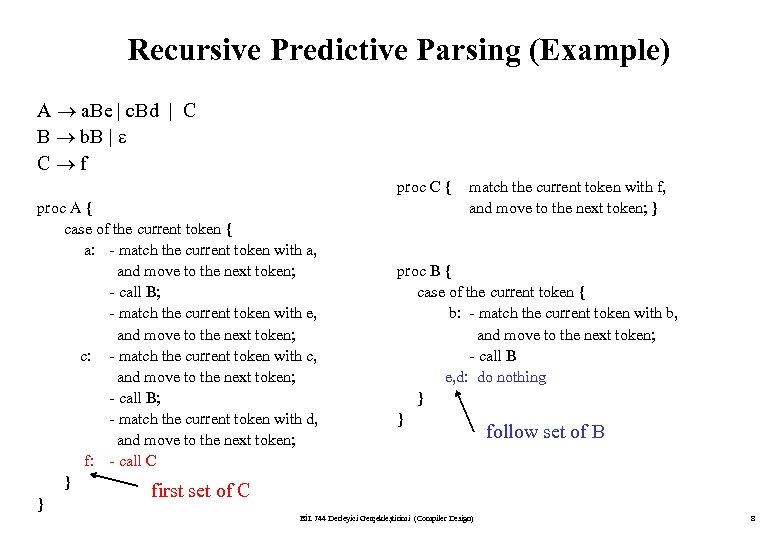Recursive Predictive Parsing (Example) A a. Be | c. Bd | C B b. B | C f proc C { proc A { case of the current token { a: - match the current token with a, and move to the next token; - call B; - match the current token with e, and move to the next token; c: - match the current token with c, and move to the next token; - call B; - match the current token with d, and move to the next token; f: - call C } first set of C } match the current token with f, and move to the next token; } proc B { case of the current token { b: - match the current token with b, and move to the next token; - call B e, d: do nothing } } BİL 744 Derleyici Gerçekleştirimi (Compiler Design) follow set of B 8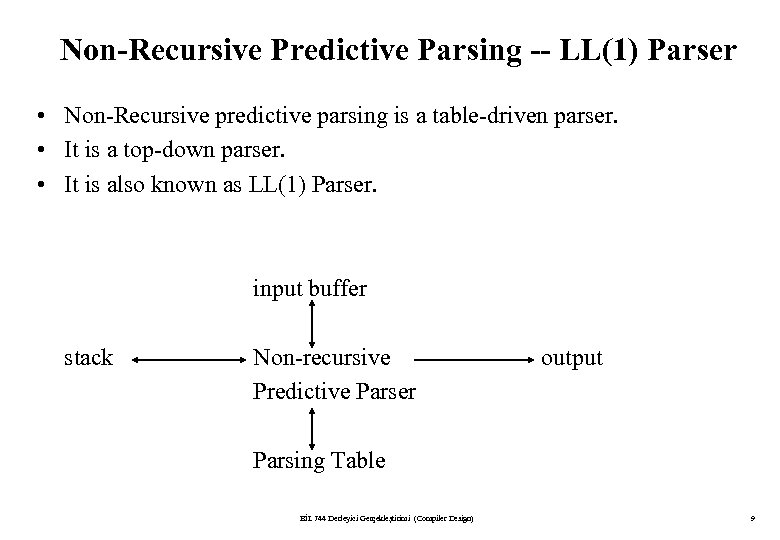Non-Recursive Predictive Parsing -- LL(1) Parser • Non-Recursive predictive parsing is a table-driven parser. • It is a top-down parser. • It is also known as LL(1) Parser. input buffer stack Non-recursive Predictive Parser output Parsing Table BİL 744 Derleyici Gerçekleştirimi (Compiler Design) 9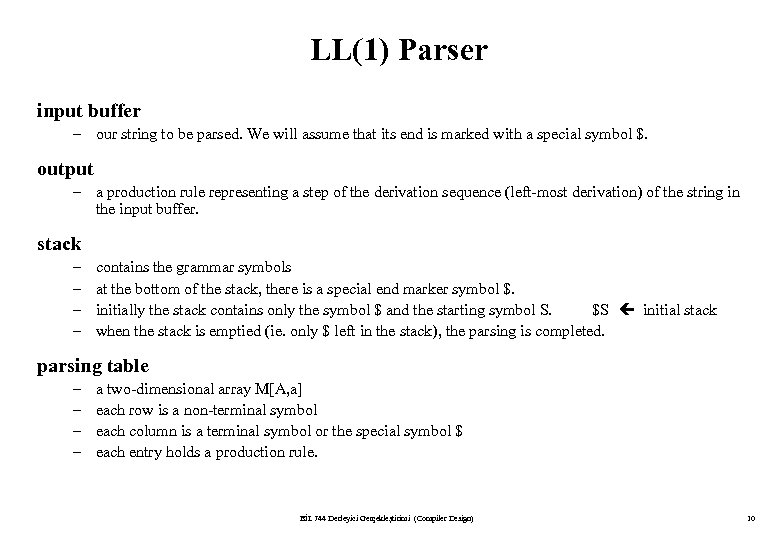LL(1) Parser input buffer – our string to be parsed. We will assume that its end is marked with a special symbol \$. output – a production rule representing a step of the derivation sequence (left-most derivation) of the string in the input buffer. stack – – contains the grammar symbols at the bottom of the stack, there is a special end marker symbol \$. initially the stack contains only the symbol \$ and the starting symbol S. \$S initial stack when the stack is emptied (ie. only \$ left in the stack), the parsing is completed. parsing table – – a two-dimensional array M[A, a] each row is a non-terminal symbol each column is a terminal symbol or the special symbol \$ each entry holds a production rule. BİL 744 Derleyici Gerçekleştirimi (Compiler Design) 10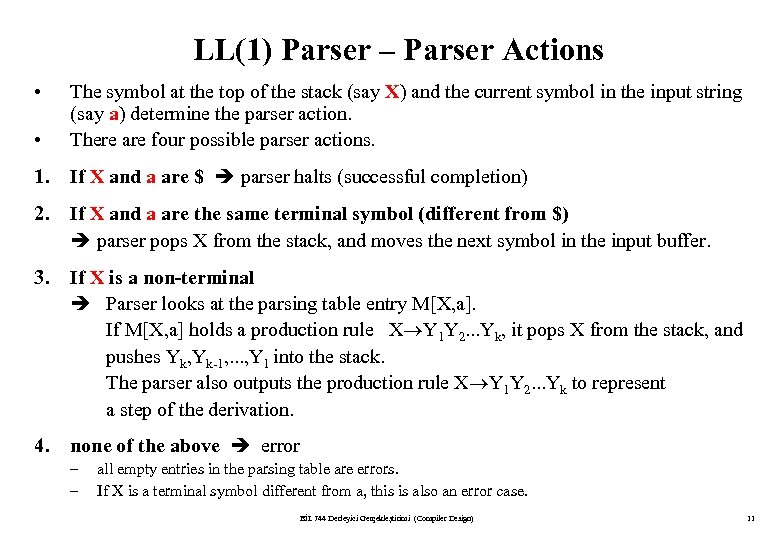LL(1) Parser – Parser Actions • • The symbol at the top of the stack (say X) and the current symbol in the input string (say a) determine the parser action. There are four possible parser actions. 1. If X and a are \$ parser halts (successful completion) 2. If X and a are the same terminal symbol (different from \$) parser pops X from the stack, and moves the next symbol in the input buffer. 3. If X is a non-terminal Parser looks at the parsing table entry M[X, a]. If M[X, a] holds a production rule X Y 1 Y 2. . . Yk, it pops X from the stack, and pushes Yk, Yk-1, . . . , Y 1 into the stack. The parser also outputs the production rule X Y 1 Y 2. . . Yk to represent a step of the derivation. 4. none of the above error – – all empty entries in the parsing table are errors. If X is a terminal symbol different from a, this is also an error case. BİL 744 Derleyici Gerçekleştirimi (Compiler Design) 11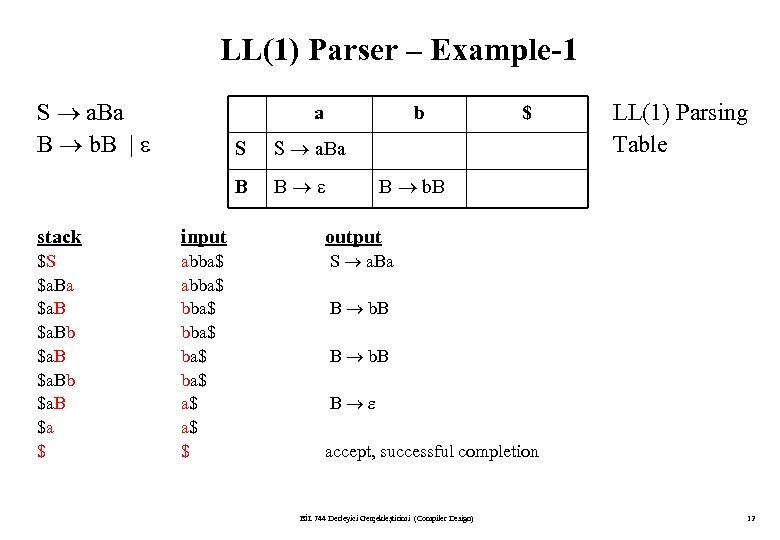LL(1) Parser – Example-1 S a. Ba B b. B | a b S S a. Ba B B \$ LL(1) Parsing Table B b. B stack input output \$S \$a. Ba \$a. Bb \$a. B \$a \$ abba\$ ba\$ a\$ a\$ \$ S a. Ba B b. B B accept, successful completion BİL 744 Derleyici Gerçekleştirimi (Compiler Design) 12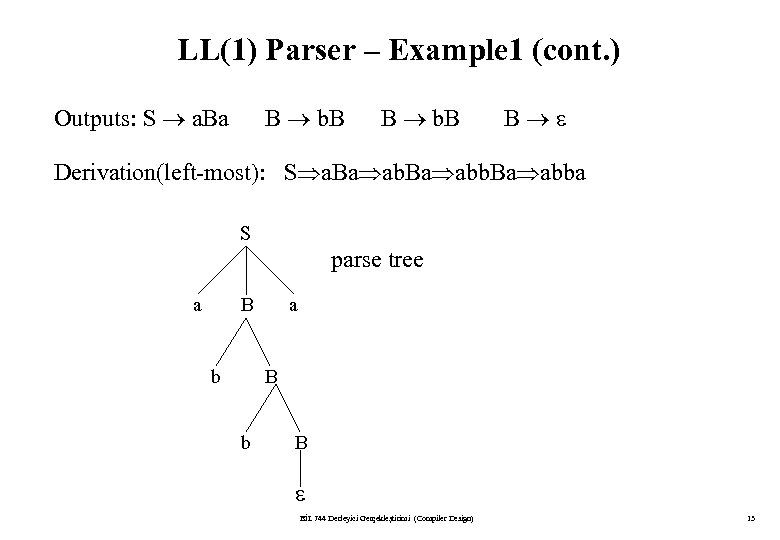LL(1) Parser – Example 1 (cont. ) Outputs: S a. Ba B b. B B Derivation(left-most): S a. Ba abba S parse tree a B b B BİL 744 Derleyici Gerçekleştirimi (Compiler Design) 13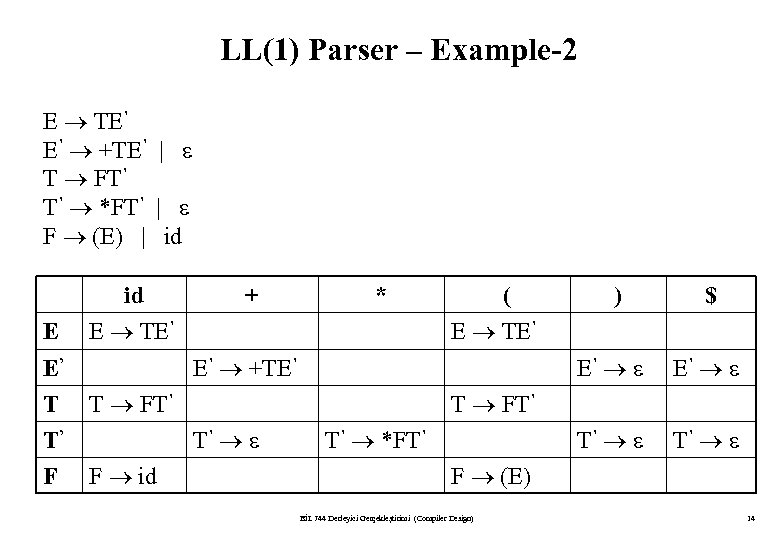LL(1) Parser – Example-2 E TE’ E’ +TE’ | T FT’ T’ *FT’ | F (E) | id E E’ T T’ F id E TE’ + * ( E TE’ E’ +TE’ \$ E’ T FT’ E’ T’ T’ T FT’ T’ F id ) T’ *FT’ F (E) BİL 744 Derleyici Gerçekleştirimi (Compiler Design) 14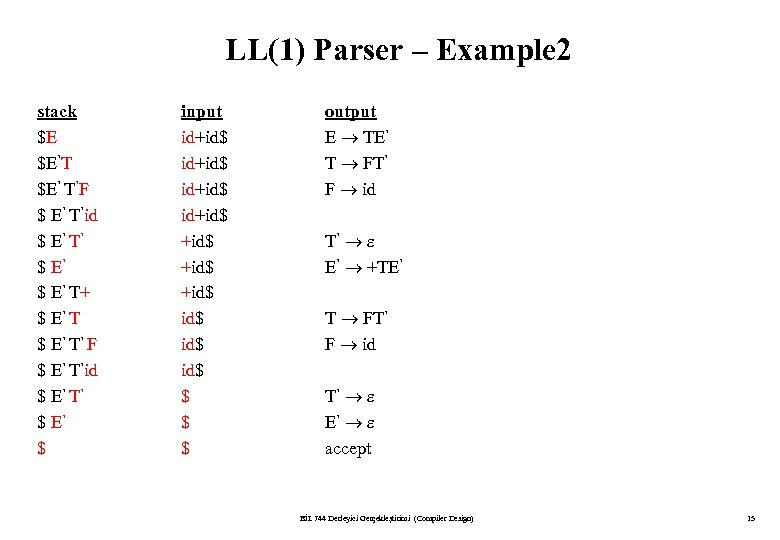LL(1) Parser – Example 2 stack \$E \$E’T \$E’ T’F \$ E’ T’id \$ E’ T’ \$ E’ T+ \$ E’ T’ F \$ E’ T’id \$ E’ T’ \$ E’ \$ input id+id\$ +id\$ id\$ \$ \$ \$ output E TE’ T FT’ F id T’ E’ +TE’ T FT’ F id T’ E’ accept BİL 744 Derleyici Gerçekleştirimi (Compiler Design) 15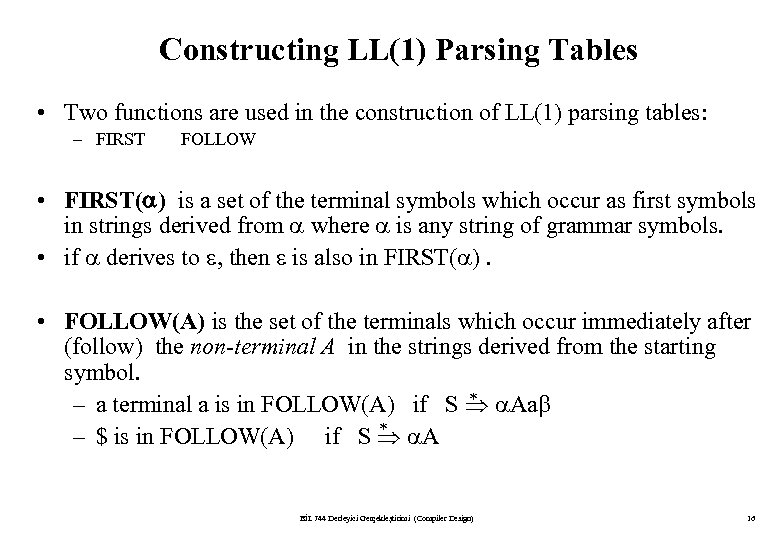Constructing LL(1) Parsing Tables • Two functions are used in the construction of LL(1) parsing tables: – FIRST FOLLOW • FIRST( ) is a set of the terminal symbols which occur as first symbols in strings derived from where is any string of grammar symbols. • if derives to , then is also in FIRST( ). • FOLLOW(A) is the set of the terminals which occur immediately after (follow) the non-terminal A in the strings derived from the starting symbol. * – a terminal a is in FOLLOW(A) if S Aa * – \$ is in FOLLOW(A) if S A BİL 744 Derleyici Gerçekleştirimi (Compiler Design) 16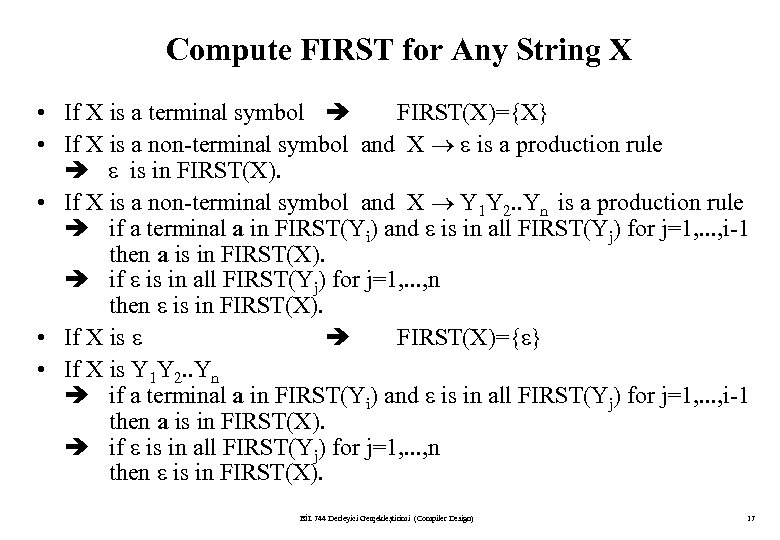Compute FIRST for Any String X • If X is a terminal symbol FIRST(X)={X} • If X is a non-terminal symbol and X is a production rule is in FIRST(X). • If X is a non-terminal symbol and X Y 1 Y 2. . Yn is a production rule if a terminal a in FIRST(Yi) and is in all FIRST(Yj) for j=1, . . . , i-1 then a is in FIRST(X). if is in all FIRST(Yj) for j=1, . . . , n then is in FIRST(X). • If X is FIRST(X)={ } • If X is Y 1 Y 2. . Yn if a terminal a in FIRST(Yi) and is in all FIRST(Yj) for j=1, . . . , i-1 then a is in FIRST(X). if is in all FIRST(Yj) for j=1, . . . , n then is in FIRST(X). BİL 744 Derleyici Gerçekleştirimi (Compiler Design) 17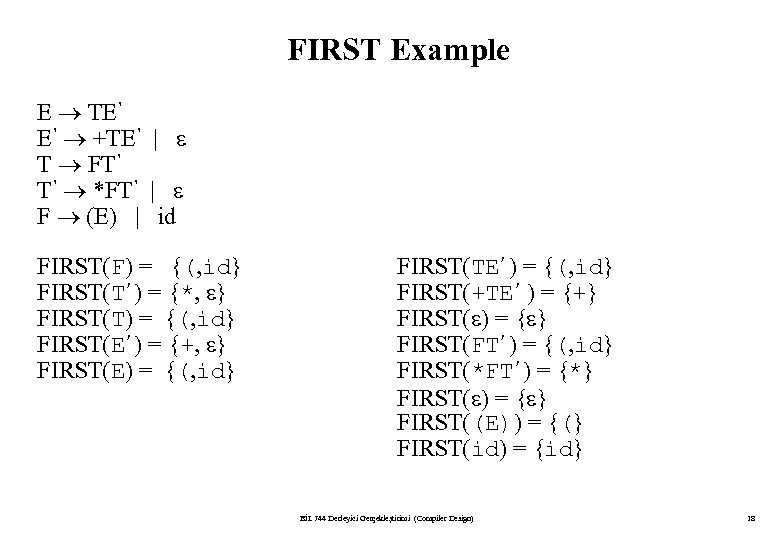FIRST Example E TE’ E’ +TE’ | T FT’ T’ *FT’ | F (E) | id FIRST(F) = {(, id} FIRST(T’) = {*, } FIRST(T) = {(, id} FIRST(E’) = {+, } FIRST(E) = {(, id} FIRST(TE’) = {(, id} FIRST(+TE’ ) = {+} FIRST( ) = { } FIRST(FT’) = {(, id} FIRST(*FT’) = {*} FIRST( ) = { } FIRST((E)) = {(} FIRST(id) = {id} BİL 744 Derleyici Gerçekleştirimi (Compiler Design) 18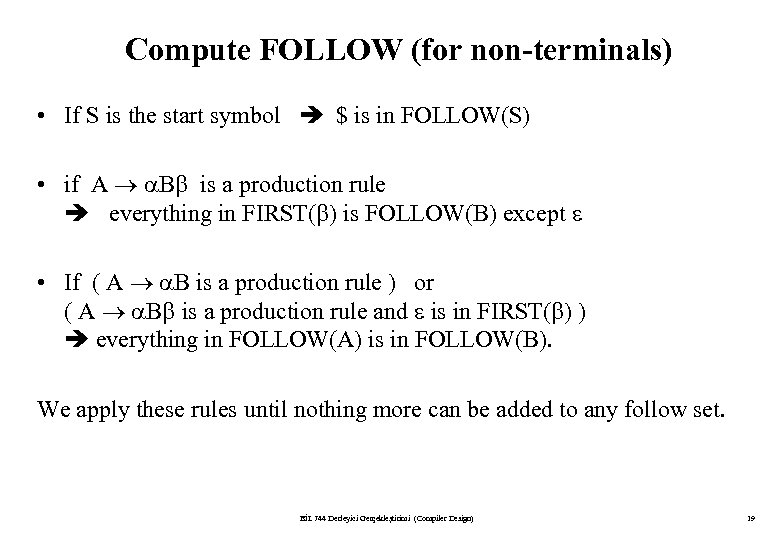Compute FOLLOW (for non-terminals) • If S is the start symbol \$ is in FOLLOW(S) • if A B is a production rule everything in FIRST( ) is FOLLOW(B) except • If ( A B is a production rule ) or ( A B is a production rule and is in FIRST( ) ) everything in FOLLOW(A) is in FOLLOW(B). We apply these rules until nothing more can be added to any follow set. BİL 744 Derleyici Gerçekleştirimi (Compiler Design) 19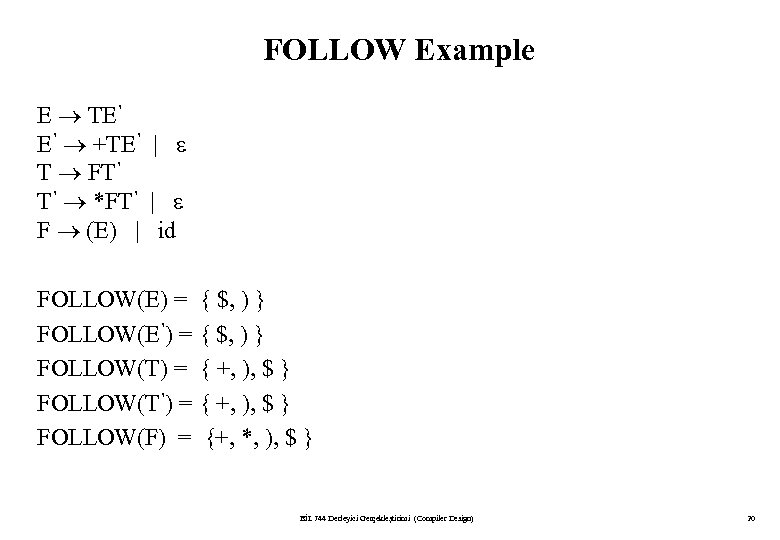FOLLOW Example E TE’ E’ +TE’ | T FT’ T’ *FT’ | F (E) | id FOLLOW(E) = { \$, ) } FOLLOW(E’) = { \$, ) } FOLLOW(T) = { +, ), \$ } FOLLOW(T’) = { +, ), \$ } FOLLOW(F) = {+, *, ), \$ } BİL 744 Derleyici Gerçekleştirimi (Compiler Design) 20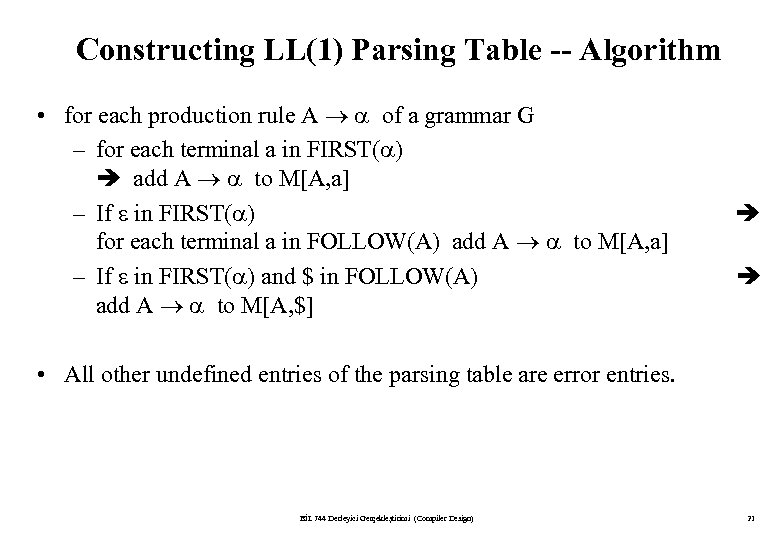Constructing LL(1) Parsing Table -- Algorithm • for each production rule A of a grammar G – for each terminal a in FIRST( ) add A to M[A, a] – If in FIRST( ) for each terminal a in FOLLOW(A) add A to M[A, a] – If in FIRST( ) and \$ in FOLLOW(A) add A to M[A, \$] • All other undefined entries of the parsing table are error entries. BİL 744 Derleyici Gerçekleştirimi (Compiler Design) 21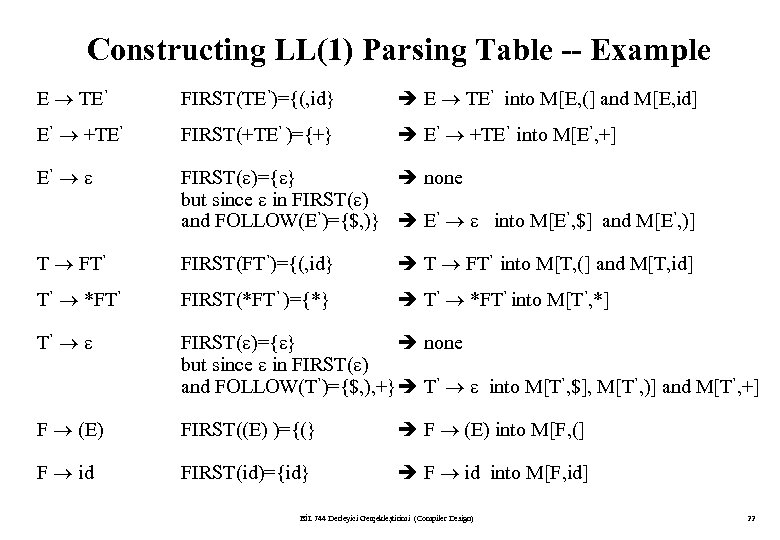Constructing LL(1) Parsing Table -- Example E TE’ FIRST(TE’)={(, id} E TE’ into M[E, (] and M[E, id] E’ +TE’ FIRST(+TE’ )={+} E’ +TE’ into M[E’, +] E’ FIRST( )={ } none but since in FIRST( ) and FOLLOW(E’)={\$, )} E’ into M[E’, \$] and M[E’, )] T FT’ FIRST(FT’)={(, id} T FT’ into M[T, (] and M[T, id] T’ *FT’ FIRST(*FT’ )={*} T’ *FT’ into M[T’, *] T’ FIRST( )={ } none but since in FIRST( ) and FOLLOW(T’)={\$, ), +} T’ into M[T’, \$], M[T’, )] and M[T’, +] F (E) FIRST((E) )={(} F (E) into M[F, (] F id FIRST(id)={id} F id into M[F, id] BİL 744 Derleyici Gerçekleştirimi (Compiler Design) 22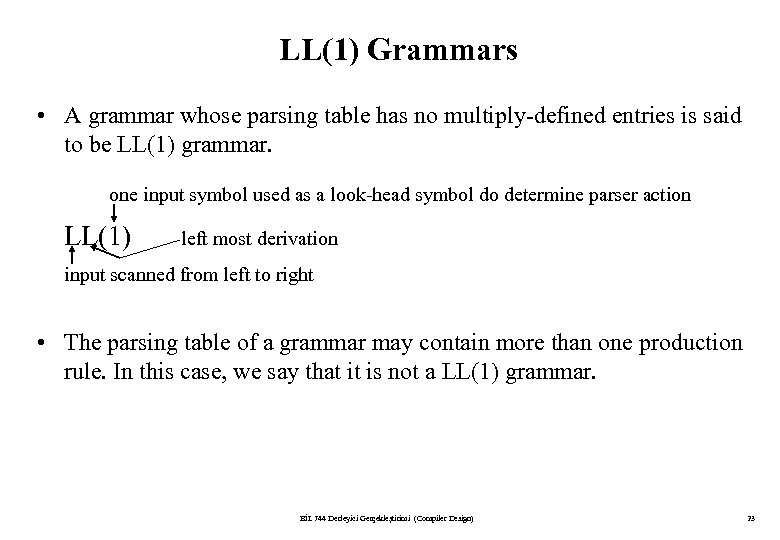LL(1) Grammars • A grammar whose parsing table has no multiply-defined entries is said to be LL(1) grammar. one input symbol used as a look-head symbol do determine parser action LL(1) left most derivation input scanned from left to right • The parsing table of a grammar may contain more than one production rule. In this case, we say that it is not a LL(1) grammar. BİL 744 Derleyici Gerçekleştirimi (Compiler Design) 23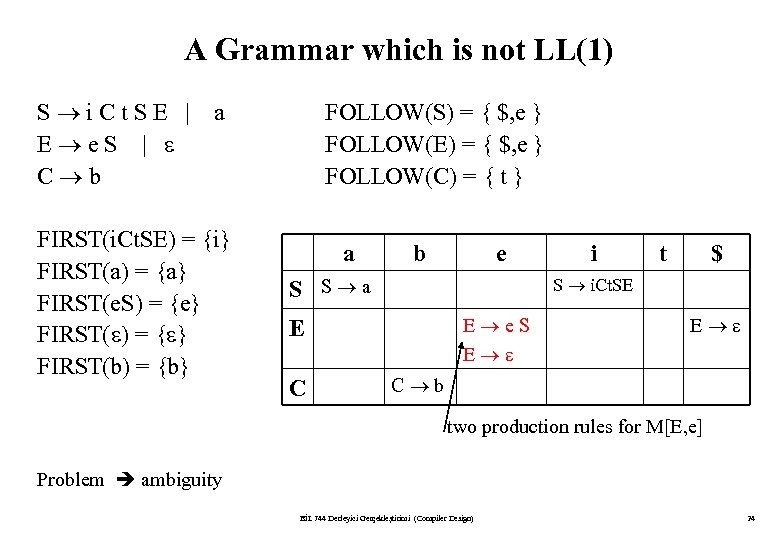A Grammar which is not LL(1) S i. Ct. SE | E e. S | C b a FIRST(i. Ct. SE) = {i} FIRST(a) = {a} FIRST(e. S) = {e} FIRST( ) = { } FIRST(b) = {b} FOLLOW(S) = { \$, e } FOLLOW(E) = { \$, e } FOLLOW(C) = { t } a b e S S a t \$ S i. Ct. SE E e. S E E C i E C b two production rules for M[E, e] Problem ambiguity BİL 744 Derleyici Gerçekleştirimi (Compiler Design) 24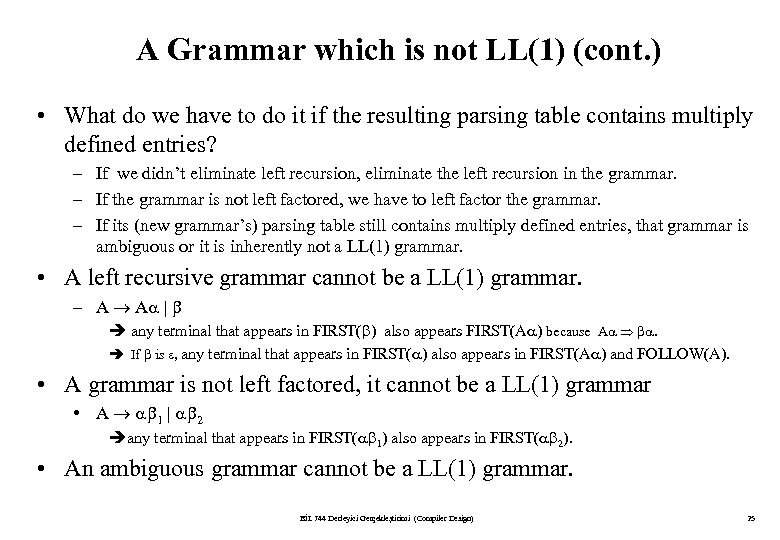A Grammar which is not LL(1) (cont. ) • What do we have to do it if the resulting parsing table contains multiply defined entries? – If we didn’t eliminate left recursion, eliminate the left recursion in the grammar. – If the grammar is not left factored, we have to left factor the grammar. – If its (new grammar’s) parsing table still contains multiply defined entries, that grammar is ambiguous or it is inherently not a LL(1) grammar. • A left recursive grammar cannot be a LL(1) grammar. – A A | any terminal that appears in FIRST( ) also appears FIRST(A ) because A . If is , any terminal that appears in FIRST( ) also appears in FIRST(A ) and FOLLOW(A). • A grammar is not left factored, it cannot be a LL(1) grammar • A 1 | 2 any terminal that appears in FIRST( 1) also appears in FIRST( 2). • An ambiguous grammar cannot be a LL(1) grammar. BİL 744 Derleyici Gerçekleştirimi (Compiler Design) 25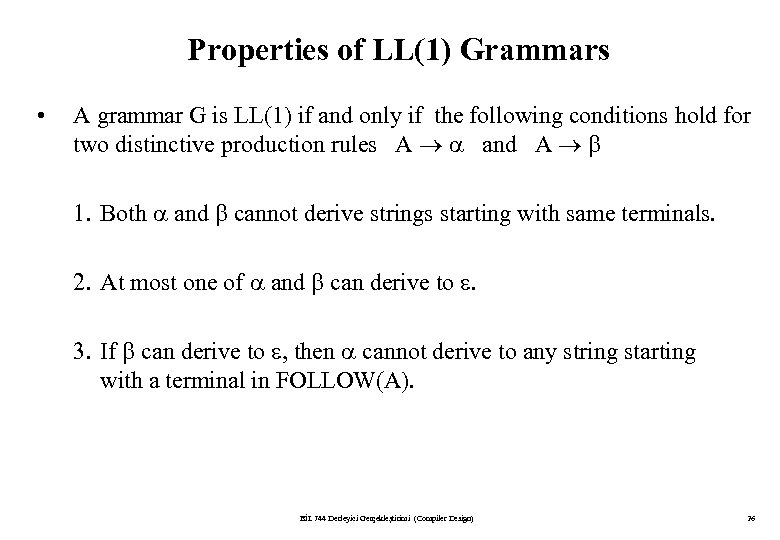Properties of LL(1) Grammars • A grammar G is LL(1) if and only if the following conditions hold for two distinctive production rules A and A 1. Both and cannot derive strings starting with same terminals. 2. At most one of and can derive to . 3. If can derive to , then cannot derive to any string starting with a terminal in FOLLOW(A). BİL 744 Derleyici Gerçekleştirimi (Compiler Design) 26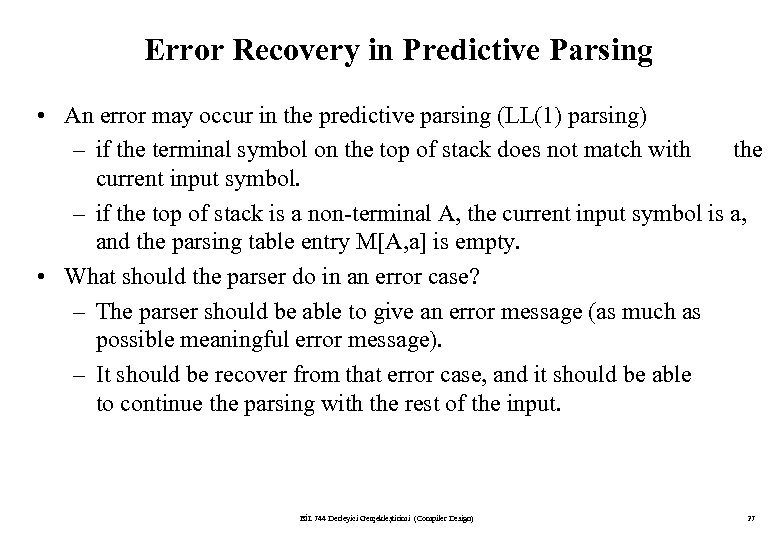Error Recovery in Predictive Parsing • An error may occur in the predictive parsing (LL(1) parsing) – if the terminal symbol on the top of stack does not match with the current input symbol. – if the top of stack is a non-terminal A, the current input symbol is a, and the parsing table entry M[A, a] is empty. • What should the parser do in an error case? – The parser should be able to give an error message (as much as possible meaningful error message). – It should be recover from that error case, and it should be able to continue the parsing with the rest of the input. BİL 744 Derleyici Gerçekleştirimi (Compiler Design) 27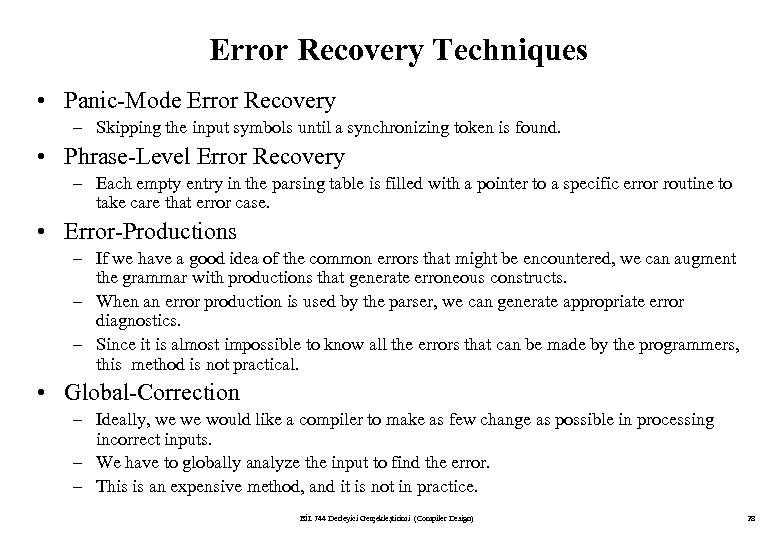Error Recovery Techniques • Panic-Mode Error Recovery – Skipping the input symbols until a synchronizing token is found. • Phrase-Level Error Recovery – Each empty entry in the parsing table is filled with a pointer to a specific error routine to take care that error case. • Error-Productions – If we have a good idea of the common errors that might be encountered, we can augment the grammar with productions that generate erroneous constructs. – When an error production is used by the parser, we can generate appropriate error diagnostics. – Since it is almost impossible to know all the errors that can be made by the programmers, this method is not practical. • Global-Correction – Ideally, we we would like a compiler to make as few change as possible in processing incorrect inputs. – We have to globally analyze the input to find the error. – This is an expensive method, and it is not in practice. BİL 744 Derleyici Gerçekleştirimi (Compiler Design) 28Panic-Mode Error Recovery in LL(1) Parsing • In panic-mode error recovery, we skip all the input symbols until a synchronizing token is found. • What is the synchronizing token? – All the terminal-symbols in the follow set of a non-terminal can be used as a synchronizing token set for that non-terminal. • So, a simple panic-mode error recovery for the LL(1) parsing: – All the empty entries are marked as synch to indicate that the parser will skip all the input symbols until a symbol in the follow set of the non-terminal A which on the top of the stack. Then the parser will pop that non-terminal A from the stack. The parsing continues from that state. – To handle unmatched terminal symbols, the parser pops that unmatched terminal symbol from the stack and it issues an error message saying that unmatched terminal is inserted. BİL 744 Derleyici Gerçekleştirimi (Compiler Design) 29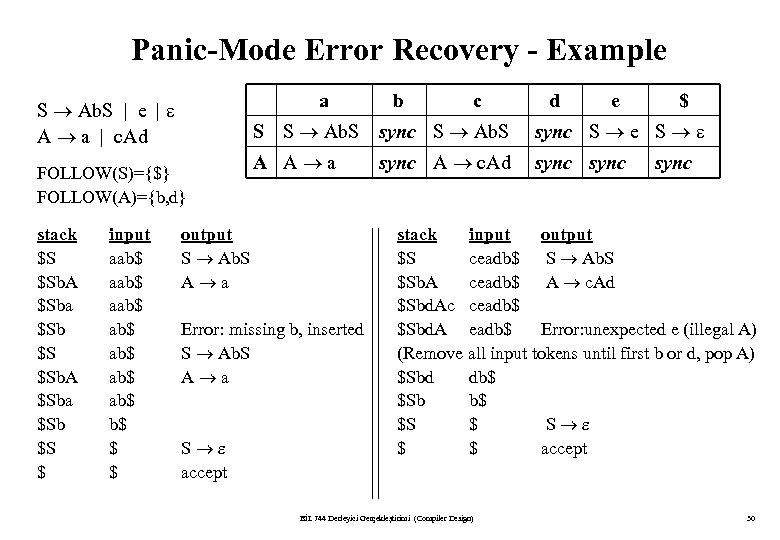Panic-Mode Error Recovery - Example a S Ab. S | e | A a | c. Ad input aab\$ ab\$ ab\$ b\$ \$ \$ c d e \$ S S Ab. S sync S Ab. S FOLLOW(S)={\$} FOLLOW(A)={b, d} stack \$S \$Sb. A \$Sba \$Sb \$S \$ b sync S e S A A a sync output S Ab. S A a Error: missing b, inserted S Ab. S A a S accept sync A c. Ad sync stack input output \$S ceadb\$ S Ab. S \$Sb. A ceadb\$ A c. Ad \$Sbd. Ac ceadb\$ \$Sbd. A eadb\$ Error: unexpected e (illegal A) (Remove all input tokens until first b or d, pop A) \$Sbd db\$ \$Sb b\$ \$S \$ S \$ \$ accept BİL 744 Derleyici Gerçekleştirimi (Compiler Design) 30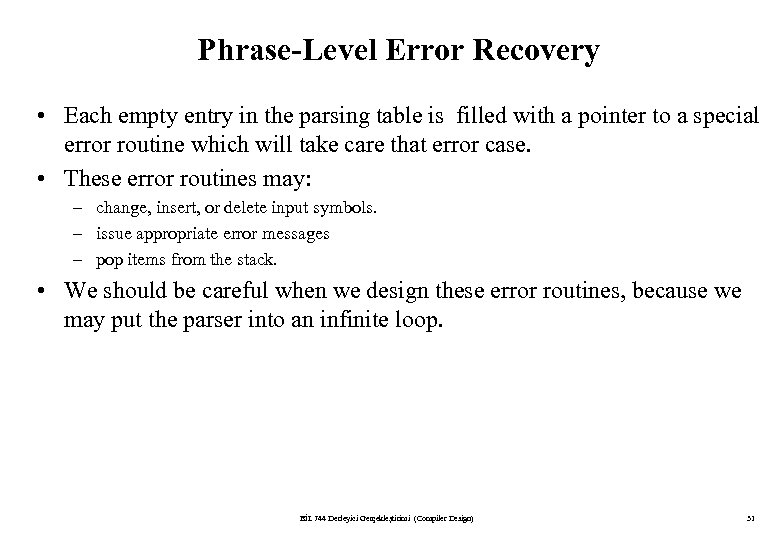Phrase-Level Error Recovery • Each empty entry in the parsing table is filled with a pointer to a special error routine which will take care that error case. • These error routines may: – change, insert, or delete input symbols. – issue appropriate error messages – pop items from the stack. • We should be careful when we design these error routines, because we may put the parser into an infinite loop. BİL 744 Derleyici Gerçekleştirimi (Compiler Design) 31